# 深入浅出讲解FOC算法与SVPWM技术

0.前言

### 0.1 什么是FOC？​

FOC（Field-Oriented Control），直译是磁场定向控制，也被称作矢量控制（VC，Vector Control），是目前无刷直流电机（BLDC）和永磁同步电机（PMSM）高效控制的最优方法之一。FOC旨在通过精确地控制磁场大小与方向，使得电机的运动转矩平稳、噪声小、效率高，并且具有高速的动态响应。

### 0.2 FOC驱动器和无刷电调的区别​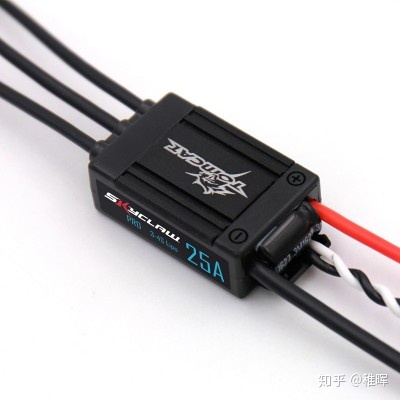FOC的优势：

1. 低转速下控制
由于控制原理的区别，无刷电调只能控制电机工作在高转速下，低速下很难控制；而FOC控制器则完全没有这个限制，不论在什么转速下都可以实现精确控制。
2. 电机换向
同上面的理由，由于无感电调无法反馈转子位置，因此很难实现电机正反转的换向；而FOC驱动器的换向性能极其优秀，最高转速下正反转切换可以非常顺畅；此外FOC还可以以能量回收的形式进行刹车控制。
3. 力矩控制
普通电调都只能控制电机转速，而FOC可以进行电流（力矩）、速度、位置三个闭环控制。
4. 噪音
FOC驱动器的噪音会比电调小很多，原因是普通电调采用方波驱动，而FOC是正弦波。

1. 兼容性
电调驱动不同的BLDC不需要进行参数整定，而FOC需要。
2. 算法复杂度
电调的算法实现更简单，运算量少，很适合需要提高带宽的超高转速电机。
3. 成本
电调的成本比FOC低很多。

## 1.从电机原理说起​

### 1.1 一些基础知识​

1. 左手定则
用于判断导线在磁场中受力的方向：伸开左手，使拇指与其他四指垂直且在一个平面内，让磁感线从手心流入，四指指向电流方向，大拇指指向的就是安培力方向（即导体受力方向）。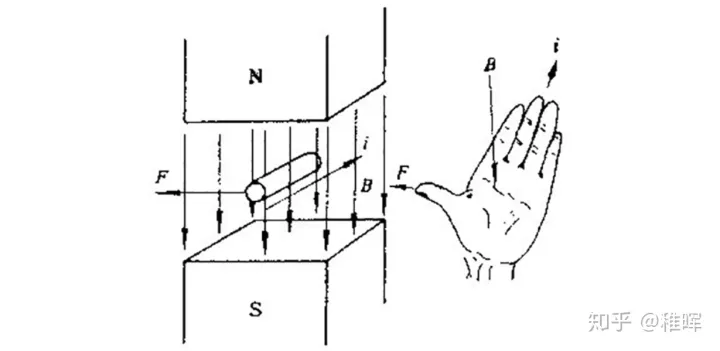1. 右手定则
伸开右手，使大拇指跟其余四个手指垂直并且都跟手掌在一个平面内，把右手放入磁场中，让磁感线垂直穿入手心，大拇指指向导体运动方向，则其余四指指向感生电动势的方向。也就是切割磁感线的导体会产生反电动势，实际上通过反电动势定位转子位置也是普通无感电调工作的基础原理之一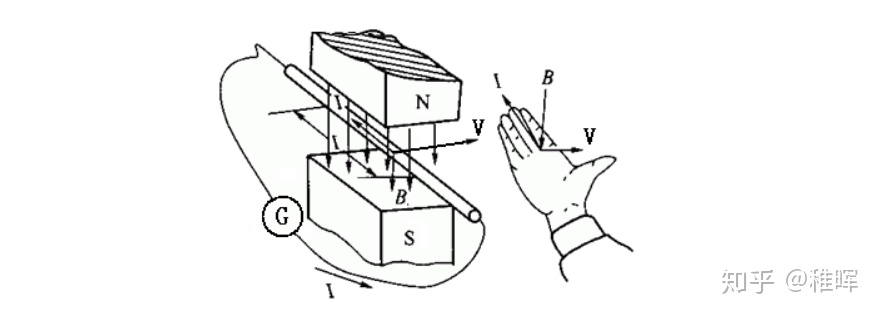1. 右手螺旋定则（即安培定则）
用于判断通电线圈判断极性：用右手握螺线管，让四指弯向螺线管中电流方向，大拇指所指的那端就是螺线管的N极。直线电流的磁场的话，大拇指指向电流方向，另外四指弯曲指的方向为磁感线的方向。1. PWM（脉冲宽度调制）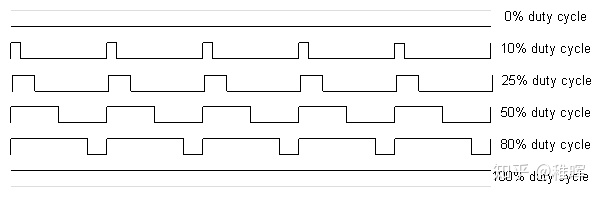不同占空比的PWM波形

### 1.2 无刷电机原理​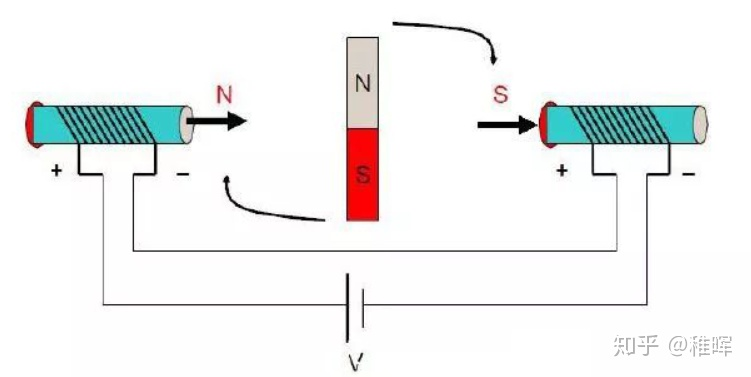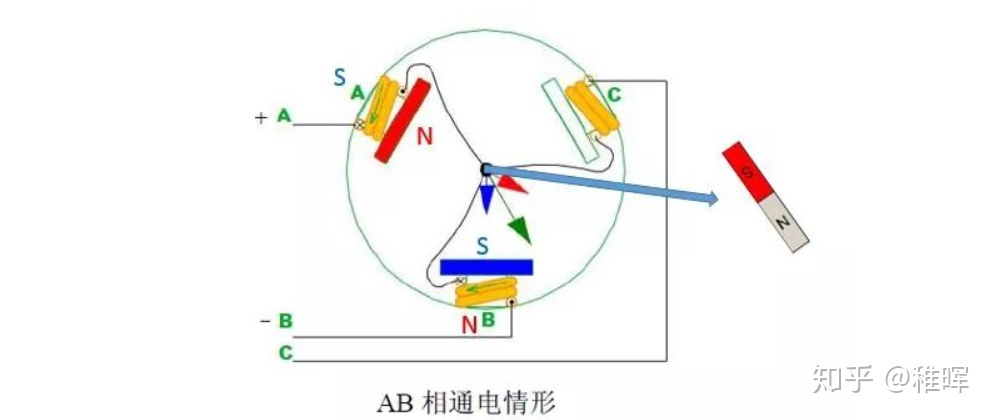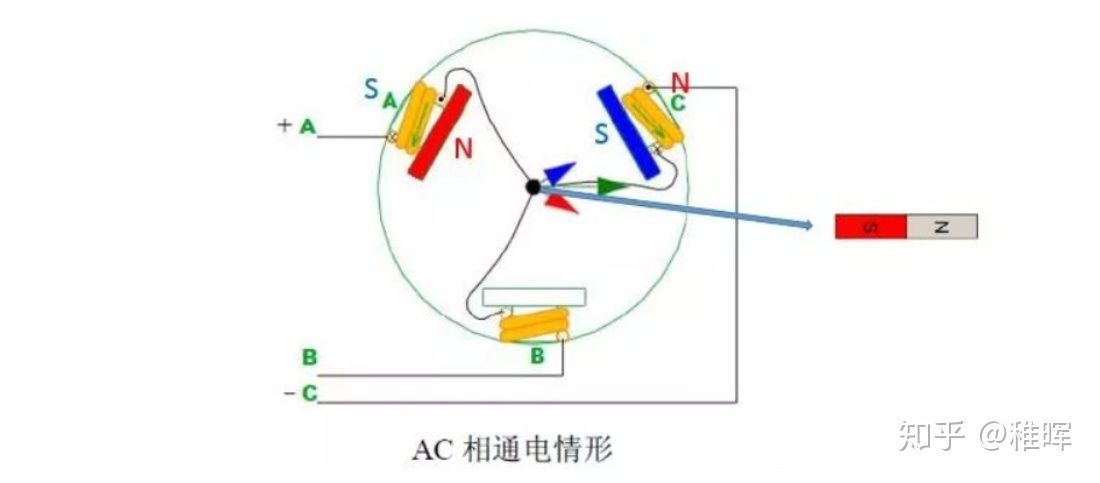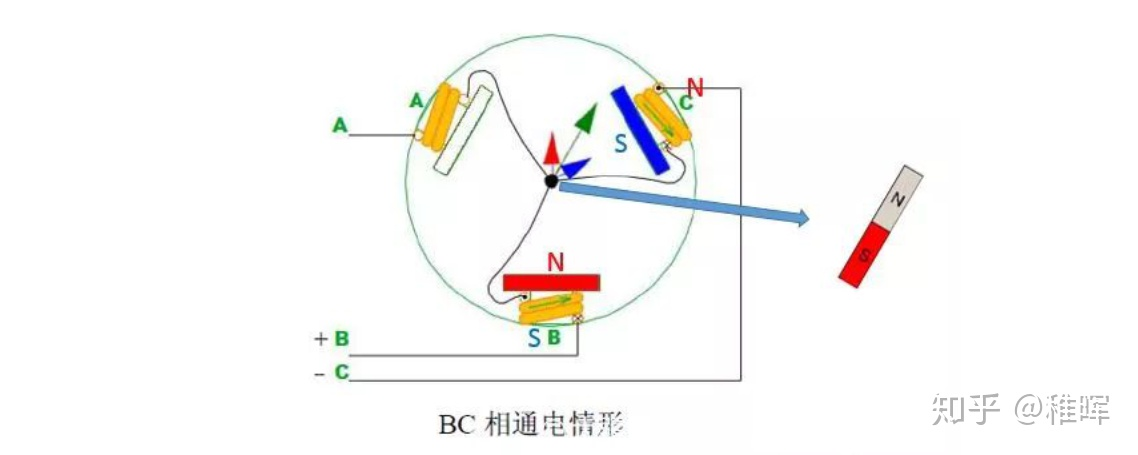...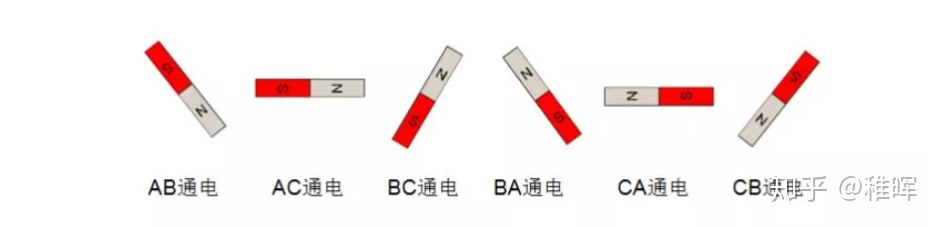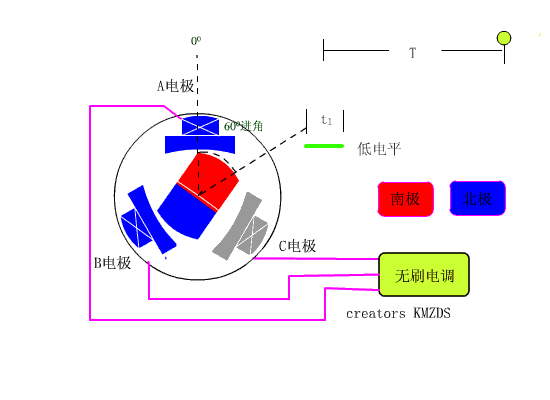## 1.3 关于BLDC和PMSM的区别​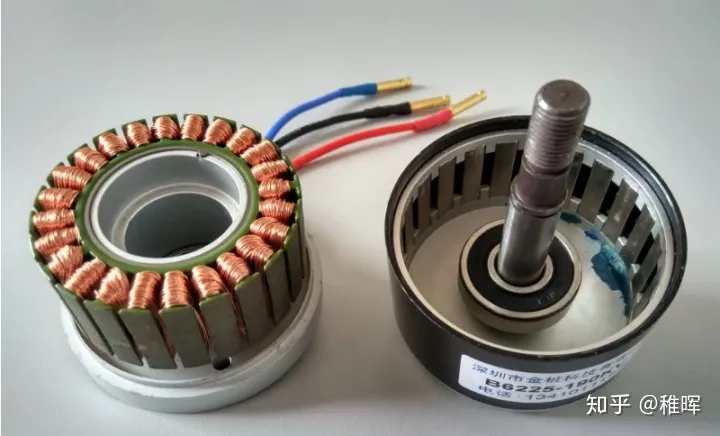### 1.3 驱动电路实现​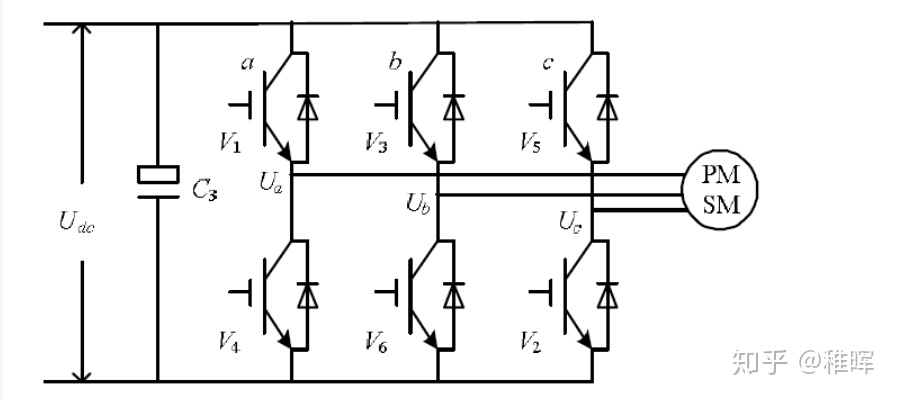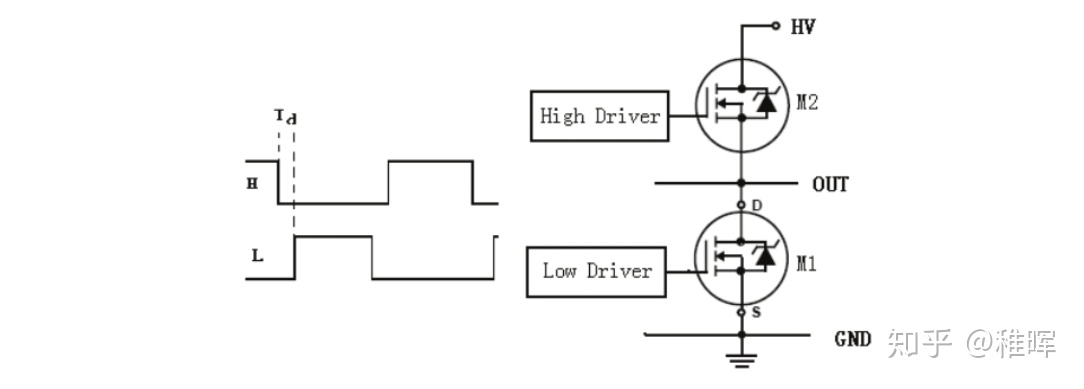三相逆变驱动电路

• 上桥开通下桥关断定义为状态1
• 上桥关断下桥开通定义为状态0

### 1.4 旋转的三相电机波形​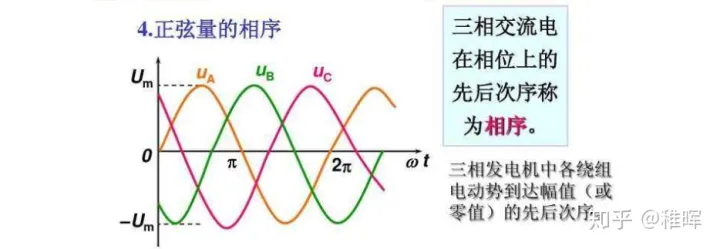## 2.FOC控制原理​

ok基础知识铺垫完毕，终于要进入正题啦~

...

### 2.1 FOC算法的Pipeline​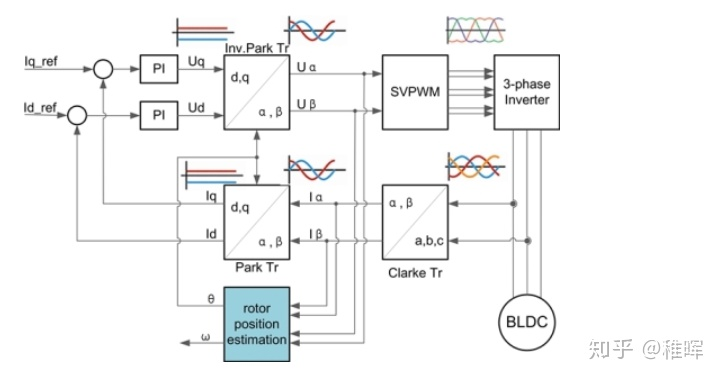1. 对电机三相电流进行采样得到 I_a,I_b,I_c
2. 将 I_a,I_b,I_c 经过`Clark变换`得到 I_{\alpha},I_{\beta}
3. 将 I_{\alpha},I_{\beta} 经过`Park变换`得到 I_q,I_d
4. 计算 I_q,I_d 和其设定值 I_q\_ref,I_d\_ref 的误差
5. 将上述误差输入两个PID（只用到PI）控制器，得到输出的控制电压 U_q,U_d
6. 将 U_q,U_d 进行`反Park变换`得到 U_{\alpha},U_{\beta}
7. 用 U_{\alpha},U_{\beta} 合成电压空间矢量，输入`SVPWM模块`进行调制，输出该时刻三个半桥的状态编码值（前文有提到）
8. 按照前面输出的编码值控制三相逆变器的MOS管开关，驱动电机
9. 循环上述步骤

### 2.2 Clark变换与Park变换​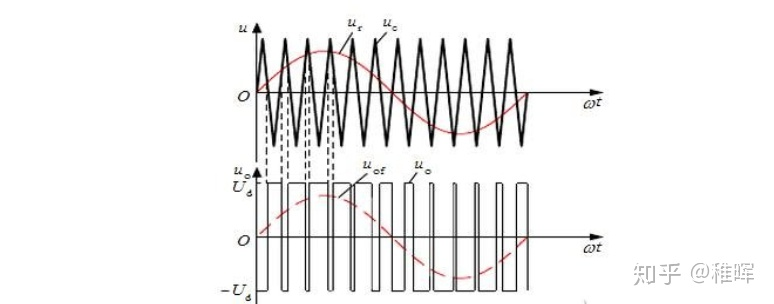### Clark变换​

I_a+I_b+I_c=0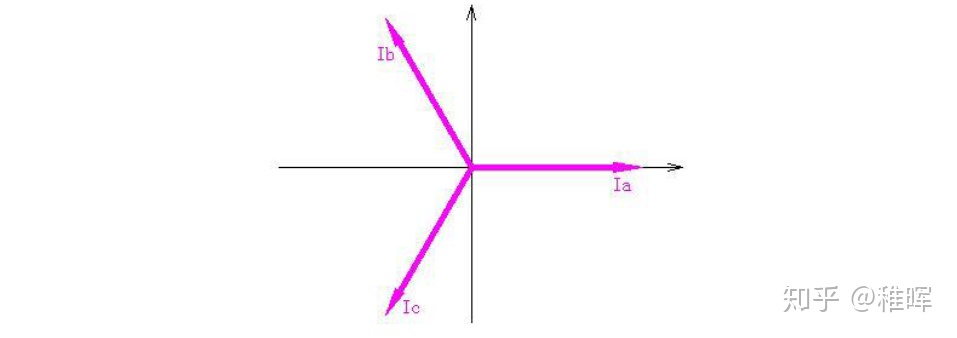\$\$ \begin{cases}I_{\alpha}=I_a-cos(\frac{2\pi}3)I_b-cos(\frac{2\pi}3)I_c\\I_{\beta}=sin(\frac{2\pi}3)I_b-sin(\frac{2\pi}3)I_c\end{cases} \$\$

\$\$ \begin{bmatrix}I_{\alpha}\\I_{\beta} \end{bmatrix}=\begin{bmatrix}1 & -\frac12 & -\frac12 &\\ 0 & \frac{\sqrt3}2 & -\frac{\sqrt3}2 \end{bmatrix} \begin{bmatrix}I_a\\I_b\\I_c \end{bmatrix} \$\$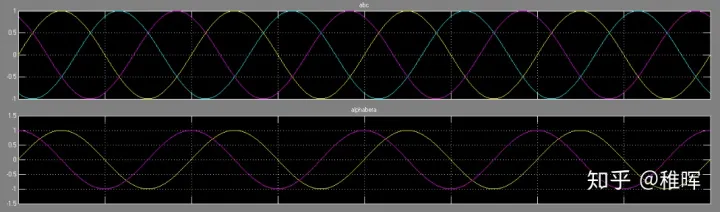### Park变换​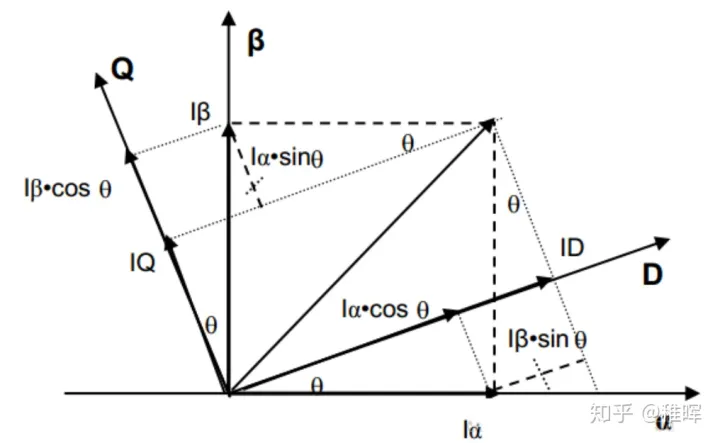\begin{cases}I_d=I_{\alpha}cos(\theta)+I_{\beta}sin(\theta)\\I_q=-I_{\alpha}sin(\theta)+I_{\beta}cos(\theta)\end{cases}

\begin{bmatrix}I_d\\I_q \end{bmatrix}=\begin{bmatrix}cos{\theta} & sin{\theta} &\\ -sin{\theta} & cos{\theta}\end{bmatrix} \begin{bmatrix}I_{\alpha}\\I_{\beta} \end{bmatrix}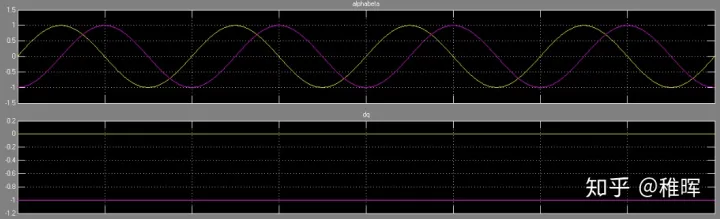Park变换前后的波形

### 2.3 PID控制​

PID（比例、积分、微分）控制是啥这篇文章就不多讲解了，基础中的基础，也有大把文章做介绍的，不熟悉的可以自行搜索相关资料。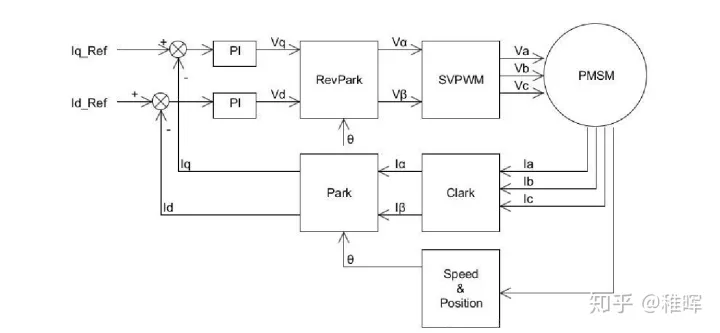PID电流环• 其中 I_q 是我们需要的，代表了期望的力矩输出
• 而 I_d 是我们不需要的，我们希望尽可能把它控制为0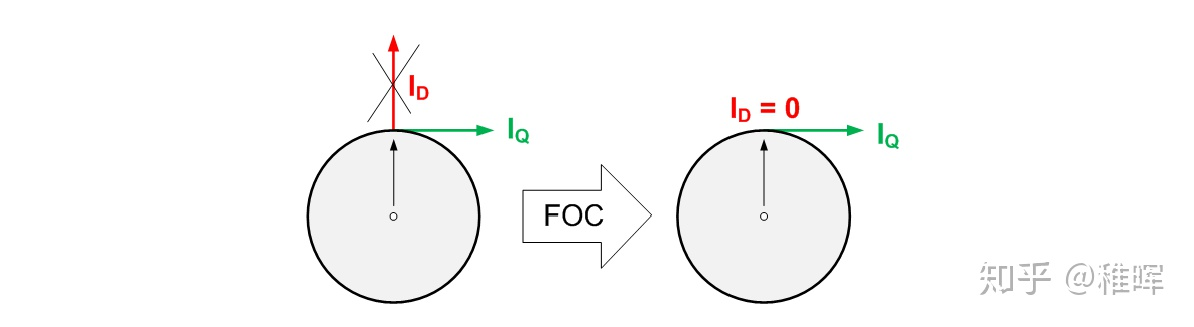FOC的控制目标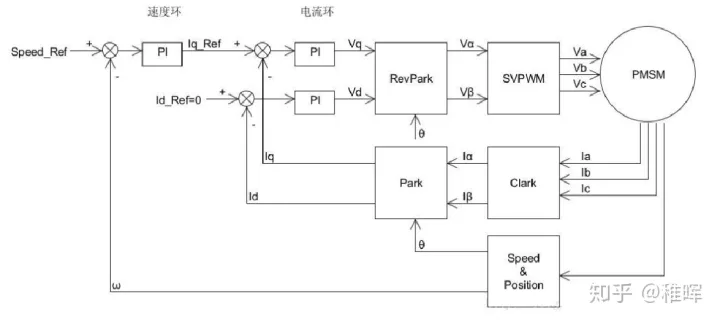速度-电流双环控制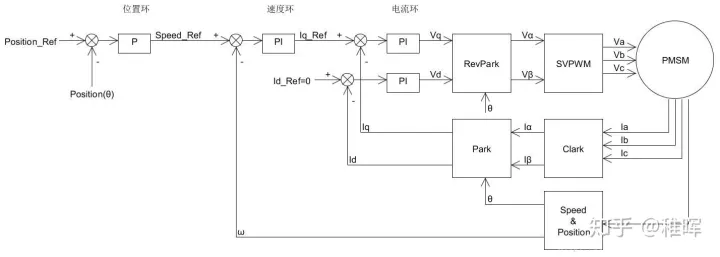位置-速度-电流三环控制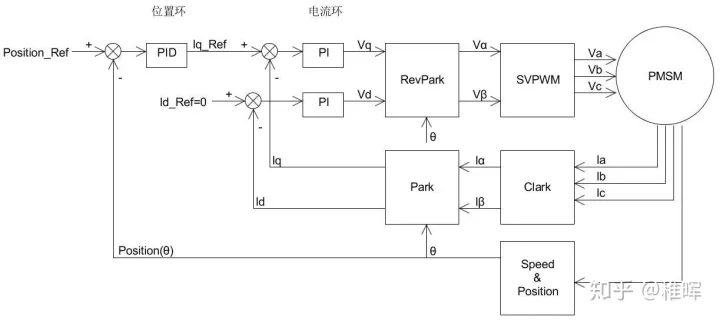位置-电流双闭环控制

### 2.4 空间电压矢量​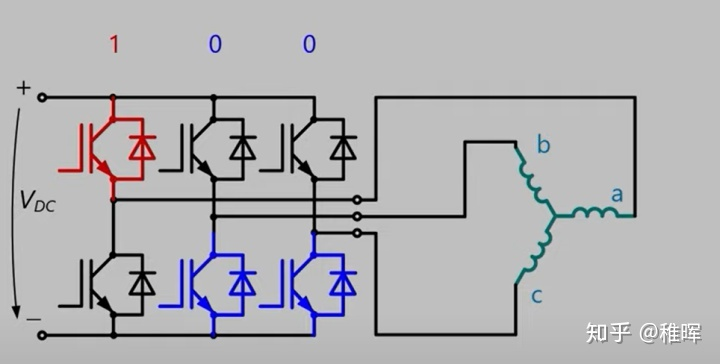U_a=U_A-U_N=\frac 23U_{dc}\\ U_b=U_B-U_N=-\frac 13U_{dc}\\ U_c=U_C-U_N=-\frac 13U_{dc}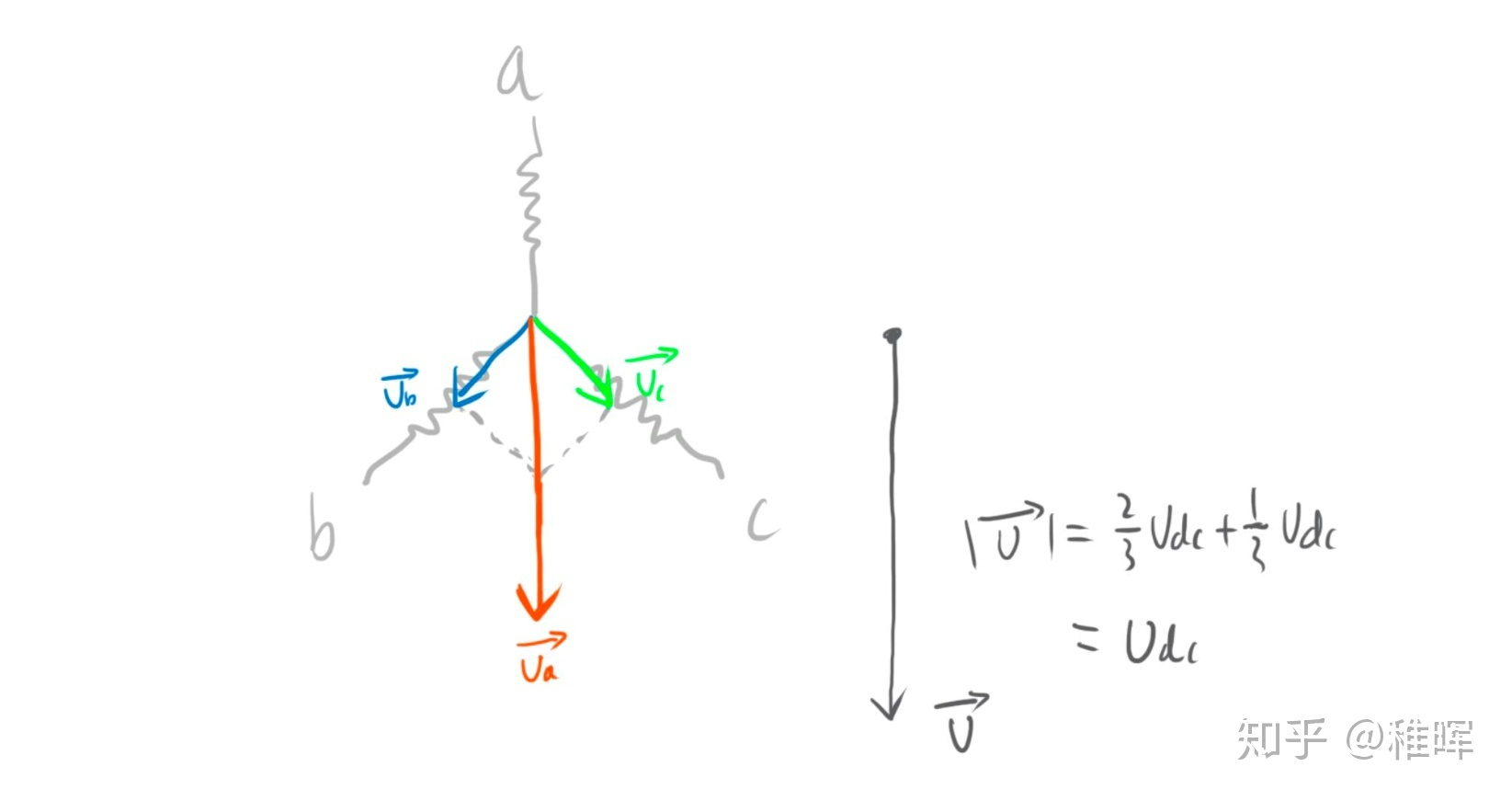\begin{cases}U_A(t)=U_{dc}cos(2\pi ft)\\U_B(t)=U_{dc}cos(2\pi ft-\frac23\pi)\\U_C(t)=U_{dc}cos(2\pi ft+\frac23\pi) \end{cases}

S_x= \begin{cases} 1,\ 上桥臂导通\\ 0,\ 下桥臂导通 \end{cases}

(S_a,S_b,S_c) 的全部可能组合共有8个，包括 6个非零矢量 ：

U_1(001)、U_2(010)、U_3(011)、U_4(100)、U_5(101)、U_6(110)

U_0(000)、U_7(111)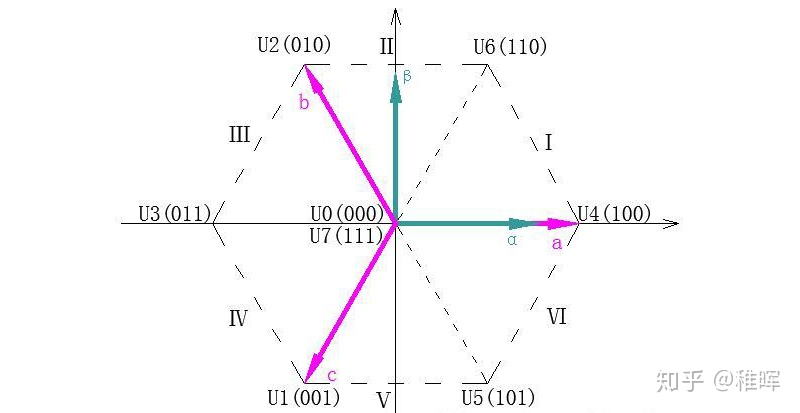ABC坐标系和αβ坐标系下的矢量表示

### 2.5 SVPWM技术​

\int_{0}^{T}U_{ref}dt=\int_{0}^{T_x}U_xdt+\int_{T_x}^{T_x+T_y}U_ydt+\int_{T_x+T_y}^{T}U_{0}^{*}dt

U_{ref}\cdot T=U_{x}\cdot T_x+U_{y}\cdot T_y+U_{0}^{*}\cdot T_{0}^{*}

U_x 和 U_y 分别是用于合成 U_{ref} 的两个空间电压矢量，也就是上面说的6个基向量中的两个，至于是哪两个？这跟 U_{ref} 所在的扇区有关，比如 U_{ref} 在Ⅰ扇区，那么 U_x 和 U_y 就是 U_4 和 U_6 ； T_x 和 T_y 就是在一个周期T中 U_x 和 U_y 所占的时间。

U_{0}^{*} 指的是两个零矢量，可以是 U_0 也可以是 U_7 ，零矢量的选择比较灵活，通过合理地配置零矢量可以让空间电压矢量的切换更平顺，后面会做说明。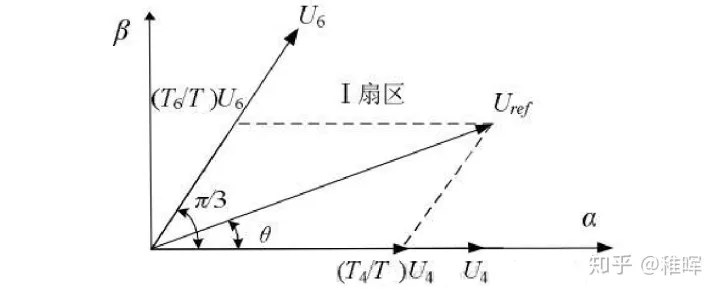\frac{\left| U_{ref} \right|}{sin\frac{2\pi}3}=\frac{\left| \frac{T_6}T \cdot U_6 \right|}{sin\theta}=\frac{\left| \frac{T_4}T \cdot U_4 \right|}{sin(\frac {\pi}3-\theta)}

\begin{cases}T_4=mTsin(\frac{\pi}3-\theta)\\T_6=mTsin\theta\end{cases}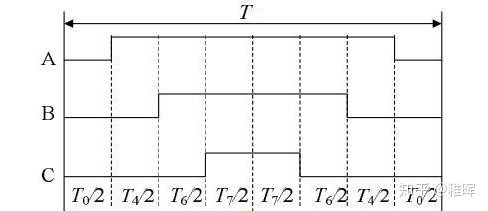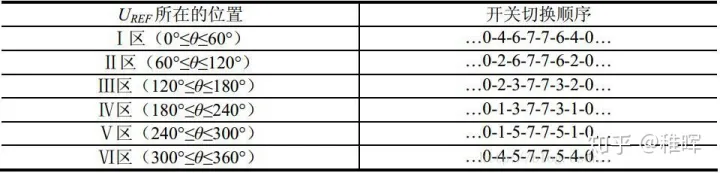## 总结​

FOC是个强大的控制方法，通过对电机的“像素级”控制，可以实现很多应用，因为可以做“力控”，FOC是很多机器人驱动单元的基础部件，比如：MIT Mini Cheetah四足机器人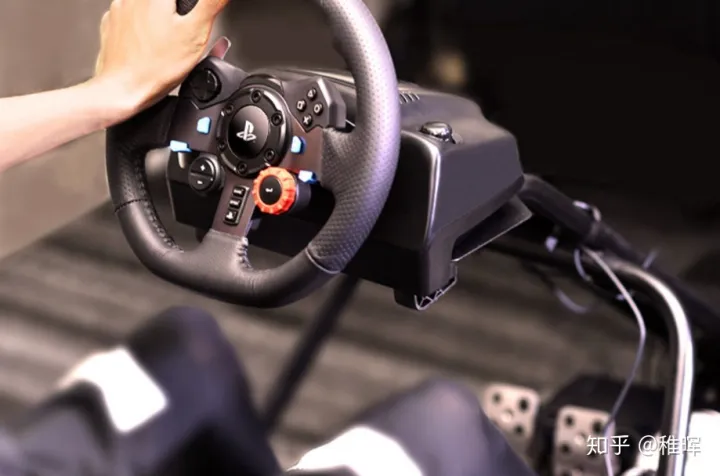罗技的力回馈方向盘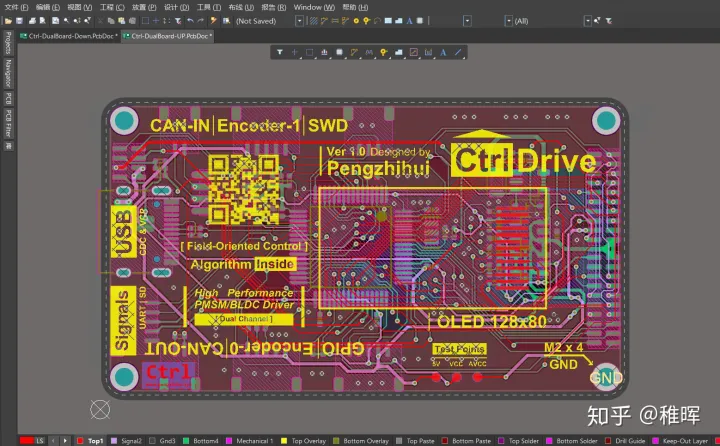逻辑控制板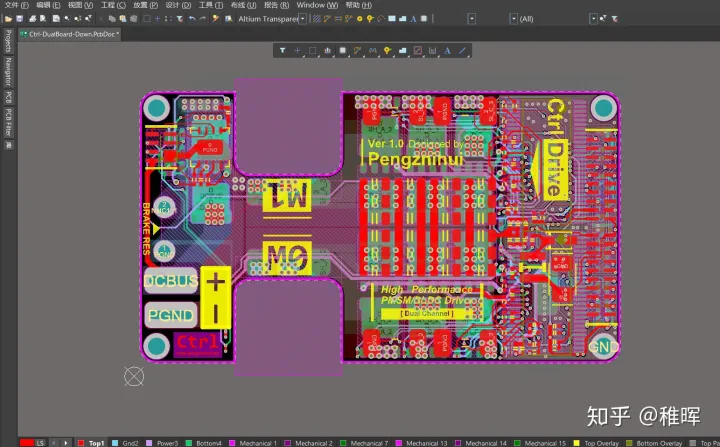功率输出板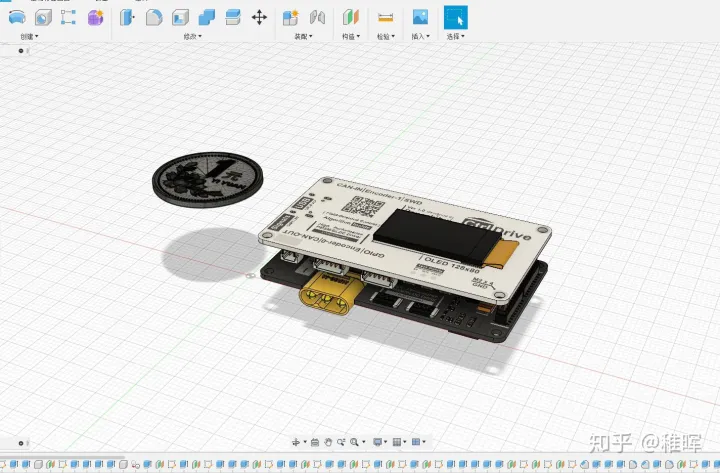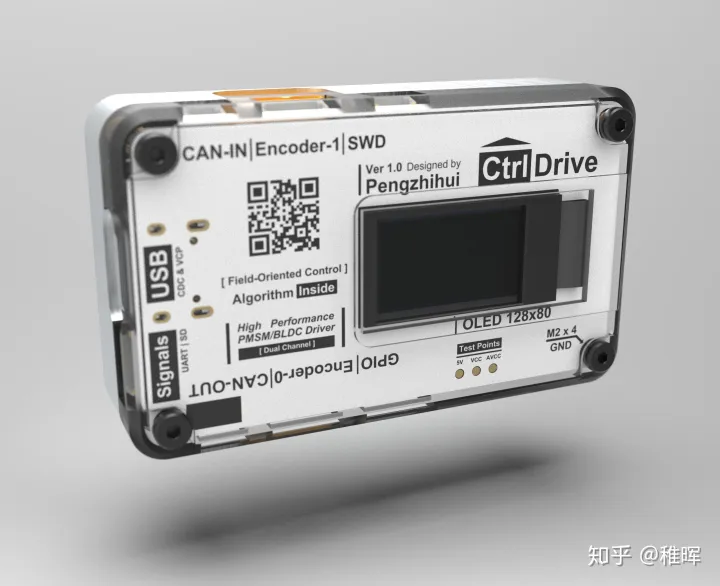## 2020.9.5更新，FOC驱动器做好啦~​

https://www.zhihu.com/zvideo/1285520312503443456

## 参考​

https://en.wikipedia.org/wiki/FOC

https://blog.csdn.net/hducollins/article/details/79260176

https://zhuanlan.zhihu.com/p/72091265

《现代电机控制技术》-王成元

《小型交流伺服电机控制电路设计》-石岛胜

TMC4671-Datasheet